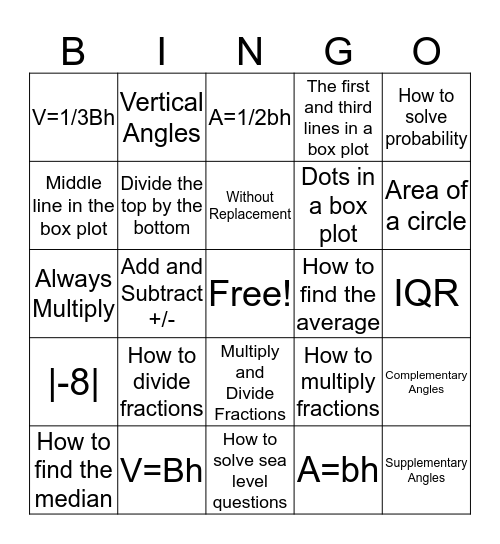# 7th Grade FSA BingoThis bingo card has a free space and 24 words: How to divide fractions, V=Bh, Without Replacement, How to find the median, How to solve probability, V=1/3Bh, Middle line in the box plot, Vertical Angles, How to find the average, Multiply and Divide Fractions, Dots in a box plot, Always Multiply, How to multiply fractions, Complementary Angles, Divide the top by the bottom, A=bh, IQR, A=1/2bh, Area of a circle, Add and Subtract +/-, Supplementary Angles, |-8|, The first and third lines in a box plot and How to solve sea level questions.

## Play Online

Share this URL with your players:For more control of your online game, create a clone of this card first.

## Probabilities

With players vying for a you'll have to call about __ items before someone wins. There's a __% chance that a lucky player would win after calling __ items.

Tip: If you want your game to last longer (on average), add more unique words/images to it.